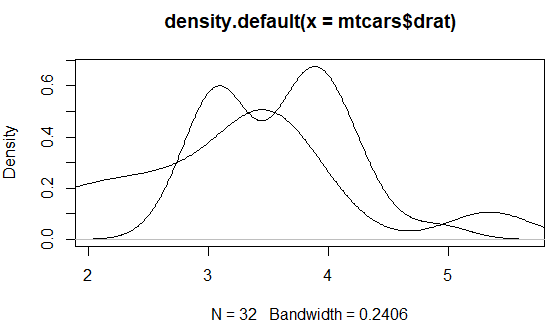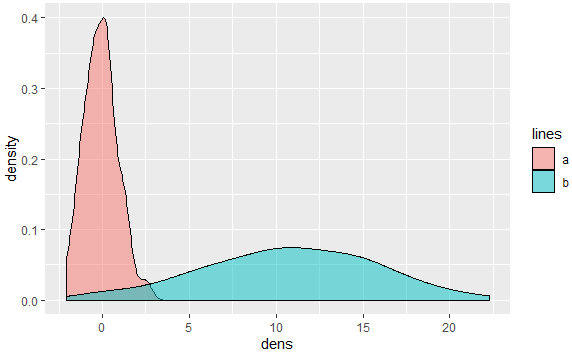5 views

I would like to overlay 2 density plots on the same device with R. How can I do that? I searched the web but I didn't find any obvious solution (I am rather new to R).

My idea would be to read data from a text file (columns) and then use

plot(density(MyData\$Column1))

well, something in this spirit...

by

To overlay density plots, you can do the following:

In base R graphics, you can use the lines() function. But make sure the limits of the first plot are suitable to plot the second one.

For example:

plot(density(mtcars\$drat))

lines(density(mtcars\$wt))

Output:In ggplot2, you can do the following:

library(ggplot2)

#Sample data

dat <- data.frame(dens = c(rnorm(100), rnorm(100, 10, 5))

, lines = rep(c("a", "b"), each = 100))

#Plot.

ggplot(dat, aes(x = dens, fill = lines)) + geom_density(alpha = 0.5)

Output: